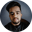Related Tags

javascript
divide by zero
js
communitycreator

# How does JavaScript handle Divide by Zero?Shubham Singh Kshatriya

### Overview

Divide by Zero is considered a special case by most programming languages. Any number can never be divided by zero because its result is indeterminate. This shot covers how JavaScript handles a Divide by Zero expression.

### How do programming languages handle it?

Most programming languages throw an exception at run time. For handling the exception, they use a try-catch block.

### How does JavaScript handle it?

JavaScript acts differently from other programming languages. When it encounters any Divide by Zero expression, it doesn’t throw an exception.

Now, the question may arise of why JavaScript behaves differently and why it doesn’t throw any exception.

The possible reasons for this are as follows:

1. JavaScript is a dynamically typed language, and it performs type-coercion.
2. Throwing exceptions at runtime is inconvenient for JavaScript.

So, how does JavaScript evaluate these expressions?

Let’s see that in the following example.

### Example

// Declare and initialize a number with 0
const num1 = 0;

// Declare and initialize a number with a positive integer
const num2 = 10;

// Declare and initialize a number with a negative integer
const num3 = -10;

// Divide by zero with a zero
console.log("Divide by zero with a zero:", num1 / 0);

// Divide by zero with a positive integer
console.log("Divide by zero with a positive integer:", num2 / 0);

// Divide by zero with a negative integer
console.log("Divide by zero with a negative integer:", num3 / 0);

### Explanation

• Line 2: We declare and initialize a variable num1 with 0.
• Line 5: We declare and initialize a variable num2 with a positive value.
• Line 8: We declare and initialize a variable num3 with a negative value.
• Line 11: We divide the variable num1 with 0, and print the output on the console.
• Line 14: We divide the variable num2 with 0, and print the output on the console.
• Line 17: We divide the variable num3 with 0, and print the output on the console.

### Output

The output of the code in JavaScript is as follows:

• Dividing the number 0 by 0 returns NaN.
• Dividing the positive number by 0 returns Infinity.
• Dividing the negative number by 0 returns -Infinity.

RELATED TAGS

javascript
divide by zero
js
communitycreator

CONTRIBUTORShubham Singh Kshatriya
RELATED COURSES

View all Courses

Keep Exploring

Learn in-demand tech skills in half the time